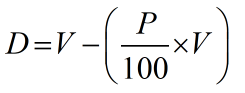Request a Tool

# Percentage Decrease Calculator

Percentage Decrease Calculator is use to calculate decrease value and its difference.

Decreased Value
0
Difference
0

#### Formula• D = Percentage decrease
• V = Value
• P = Percentage

#### Defination / Uses

The amount of a reduction, such as a wage cut or a budget cut, is referred to as a reduction. Instead of just a raw number, using a percentage to express a reduction measures the extent of the reduction in respect to the original amount.

The % reduction calculator calculates the change in percentage from one amount to a lesser amount. It's critical to know how to manually compute percent decrease using the percent decrease formula. These sections will go through these principles in further depth. As a result, you must give both an original and a new quantity. If the new quantity is smaller than the old, the change will be an increase. The difference between the two quantities is calculated and divided by the initial quantity. The value is then multiplied by a factor of 100. The % difference and percentage change calculators can also be used to do this.